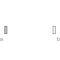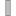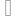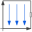# PartialForce

Base model for force elements (provide frame_b.f and frame_b.t in subclasses)# Information

This information is part of the Modelica Standard Library maintained by the Modelica Association.

All 3-dimensional force and torque elements should be based on this superclass. This model defines frame_a and frame_b, computes the relative translation and rotation between the two frames and calculates the cut-force and cut-torque at frame_a by a force and torque balance from the cut-force and cut-torque at frame_b. As a result, in a subclass, only the relationship between the cut-force and cut-torque at frame_b has to be defined as a function of the following relative quantities:

```  r_rel_b: Position vector from origin of frame_a to origin
of frame_b, resolved in frame_b
R_rel     : Relative orientation object to rotate from frame_a to frame_b
```

Assume that force f = {100,0,0} should be applied on the body to which this force element is attached at frame_b, then the definition should be:

```   model Constant_x_Force
extends Modelica.Mechanics.MultiBody.Interfaces.PartialForce;
equation
frame_b.f = {-100, 0, 0};
frame_b.t = zeros(3);
end Constant_x_Force;
```

Note, that frame_b.f and frame_b.t are flow variables and therefore the negative value of frame_b.f and frame_b.t is acting at the part to which this force element is connected.

# Connectors (2)

frame_a frame_bType: Frame_a Description: Coordinate system a fixed to the component with one cut-force and cut-torqueType: Frame_b Description: Coordinate system b fixed to the component with one cut-force and cut-torque

# Components (1)

 worldType: World